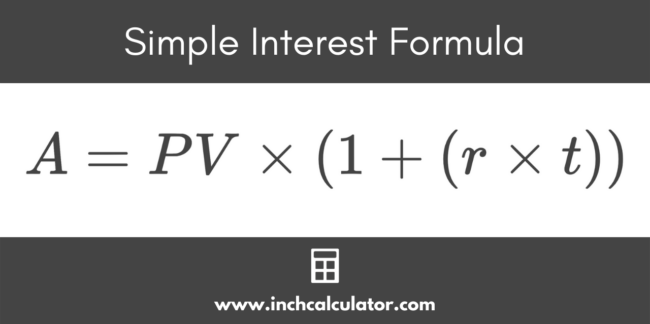# Simple Interest Calculator

Calculate the interest earned and the future value of an investment using simple interest by entering the principal, rate, and time below.

\$
years

## Future Value using Simple Interest:

 Total Value: \$1,325.00 Total Interest: \$325.00

### Balance by Year

This calculation is based on widely-accepted formulas for educational purposes only - this is not a recommendation for how to handle your finances, and it is not an offer to lend or invest. Consult with a financial professional before making financial decisions.
Learn how we calculated this below

## How to Calculate Simple Interest

Simple interest is a method for calculating interest on a loan. A simple interest calculator provides a quick and easy way to calculate the interest on a loan given the principal value, interest rate, and number of time periods.

Simple interest differs from compound interest in that interest is not paid on prior interest. Interest is only paid on the principal value, so the interest paid from simple interest should be slightly less than typical future value calculations.

### Simple Interest Formula

The simple interest formula is as follows:

A = PV × (1 + (r × t))

where:
A = amount
PV = principal value
r = interest rate
t = number of periods

The amount A is equal to the principal value PV times 1 plus the interest rate r times the number of compounding periods t.

Note that this simple interest formula can be rewritten as follows to calculate the same result:

A = PV + (PV × r × t)

This new formula breaks out the amount borrowed/lent (PV) from the interest paid/received (PV × r × t).For example, let’s say you purchased \$8,000 in bonds paying 3% simple interest over 10 years.

Let’s plug the numbers into the simple interest formula:

A = \$8,000 × (1 + (0.03 × 10 years))
A = \$8,000 × (1 + 0.3)
A = \$8,000 × 1.3
A = \$10,400

The bonds would pay you \$10,400 total over the 10 years, which is \$2,400 in interest.

You can also use our bond yield calculator to learn more about how much bonds yield over time.

### How is simple interest different from compound interest?

Simple interest uses the current principal value to calculate the interest payment for each compounding period, while compound interest uses the principal value in addition to earned interest to calculate the interest payment for each period.

So, compound interest accrues interest on previously accumulated interest, meaning more interest is earned in each compounding period. This is referred to as “interest on interest”.

### When is simple interest used?

Simple interest can be used on loans and bonds. Loans that include simple interest include mortgages, credit cards, and student loans, to name a few.

If you purchase bonds from the government or a corporation, you will receive simple interest.

A savings account is an example of when simple interest is not used. Assuming you leave prior interest payments in the account, you will receive interest on the original value plus the prior interest payments. This is compounding interest.

Simple interest is used when you pay back a loan because you only pay interest on the current value of the loan, not the previous interest that has accrued. Another example of simple interest is when you lend money to the government–you receive interest only on the original amount invested, and not on each previous interest payment you have received.

### Is simple interest linear or exponential?

The graph of the value of an investment earning simple interest will be linear because the amount of growth is the same in each compounding period.

The graph of the value of an investment earning compound interest will be exponential because the amount of growth is more in each compounding period as the interest is accrued on previous interest in addition to the principal.